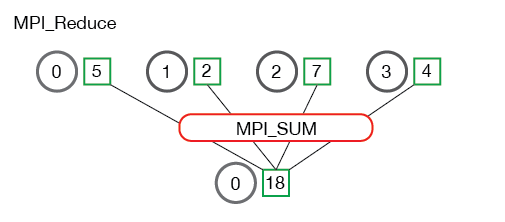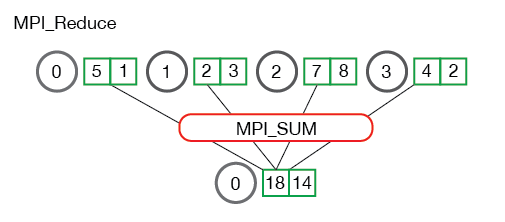# MPI Reduce and Allreduce

Author: Wes Kendall

In the previous lesson, we went over an application example of using `MPI_Scatter` and `MPI_Gather` to perform parallel rank computation with MPI. We are going to expand on collective communication routines even more in this lesson by going over `MPI_Reduce` and `MPI_Allreduce`.

Note - All of the code for this site is on GitHub. This tutorial’s code is under tutorials/mpi-reduce-and-allreduce/code.

## An introduction to reduce

Reduce is a classic concept from functional programming. Data reduction involves reducing a set of numbers into a smaller set of numbers via a function. For example, let’s say we have a list of numbers `[1, 2, 3, 4, 5]`. Reducing this list of numbers with the sum function would produce `sum([1, 2, 3, 4, 5]) = 15`. Similarly, the multiplication reduction would yield `multiply([1, 2, 3, 4, 5]) = 120`.

As you might have imagined, it can be very cumbersome to apply reduction functions across a set of distributed numbers. Along with that, it is difficult to efficiently program non-commutative reductions, i.e. reductions that must occur in a set order. Luckily, MPI has a handy function called `MPI_Reduce` that will handle almost all of the common reductions that a programmer needs to do in a parallel application.

## MPI_Reduce

Similar to `MPI_Gather`, `MPI_Reduce` takes an array of input elements on each process and returns an array of output elements to the root process. The output elements contain the reduced result. The prototype for `MPI_Reduce` looks like this:

``````MPI_Reduce(
void* send_data,
void* recv_data,
int count,
MPI_Datatype datatype,
MPI_Op op,
int root,
MPI_Comm communicator)
``````

The `send_data` parameter is an array of elements of type `datatype` that each process wants to reduce. The `recv_data` is only relevant on the process with a rank of `root`. The `recv_data` array contains the reduced result and has a size of `sizeof(datatype) * count`. The `op` parameter is the operation that you wish to apply to your data. MPI contains a set of common reduction operations that can be used. Although custom reduction operations can be defined, it is beyond the scope of this lesson. The reduction operations defined by MPI include:

• `MPI_MAX` - Returns the maximum element.
• `MPI_MIN` - Returns the minimum element.
• `MPI_SUM` - Sums the elements.
• `MPI_PROD` - Multiplies all elements.
• `MPI_LAND` - Performs a logical and across the elements.
• `MPI_LOR` - Performs a logical or across the elements.
• `MPI_BAND` - Performs a bitwise and across the bits of the elements.
• `MPI_BOR` - Performs a bitwise or across the bits of the elements.
• `MPI_MAXLOC` - Returns the maximum value and the rank of the process that owns it.
• `MPI_MINLOC` - Returns the minimum value and the rank of the process that owns it.

Below is an illustration of the communication pattern of `MPI_Reduce`.In the above, each process contains one integer. `MPI_Reduce` is called with a root process of 0 and using `MPI_SUM` as the reduction operation. The four numbers are summed to the result and stored on the root process.

It is also useful to see what happens when processes contain multiple elements. The illustration below shows reduction of multiple numbers per process.The processes from the above illustration each have two elements. The resulting summation happens on a per-element basis. In other words, instead of summing all of the elements from all the arrays into one element, the ith element from each array are summed into the ith element in result array of process 0.

Now that you understand how `MPI_Reduce` looks, we can jump into some code examples.

## Computing average of numbers with MPI_Reduce

In the previous lesson, I showed you how to compute average using `MPI_Scatter` and `MPI_Gather`. Using `MPI_Reduce` simplifies the code from the last lesson quite a bit. Below is an excerpt from reduce_avg.c in the example code from this lesson.

``````float *rand_nums = NULL;
rand_nums = create_rand_nums(num_elements_per_proc);

// Sum the numbers locally
float local_sum = 0;
int i;
for (i = 0; i < num_elements_per_proc; i++) {
local_sum += rand_nums[i];
}

// Print the random numbers on each process
printf("Local sum for process %d - %f, avg = %f\n",
world_rank, local_sum, local_sum / num_elements_per_proc);

// Reduce all of the local sums into the global sum
float global_sum;
MPI_Reduce(&local_sum, &global_sum, 1, MPI_FLOAT, MPI_SUM, 0,
MPI_COMM_WORLD);

// Print the result
if (world_rank == 0) {
printf("Total sum = %f, avg = %f\n", global_sum,
global_sum / (world_size * num_elements_per_proc));
}
``````

In the code above, each process creates random numbers and makes a `local_sum` calculation. The `local_sum` is then reduced to the root process using `MPI_SUM`. The global average is then `global_sum / (world_size * num_elements_per_proc)`. If you run the reduce_avg program from the tutorials directory of the repo, the output should look similar to this.

``````>>> cd tutorials
>>> ./run.py reduce_avg
mpirun -n 4  ./reduce_avg 100
Local sum for process 0 - 51.385098, avg = 0.513851
Local sum for process 1 - 51.842468, avg = 0.518425
Local sum for process 2 - 49.684948, avg = 0.496849
Local sum for process 3 - 47.527420, avg = 0.475274
Total sum = 200.439941, avg = 0.501100
``````

Now it is time to move on to the sibling of `MPI_Reduce` - `MPI_Allreduce`.

## MPI_Allreduce

Many parallel applications will require accessing the reduced results across all processes rather than the root process. In a similar complementary style of `MPI_Allgather` to `MPI_Gather`, `MPI_Allreduce` will reduce the values and distribute the results to all processes. The function prototype is the following:

``````MPI_Allreduce(
void* send_data,
void* recv_data,
int count,
MPI_Datatype datatype,
MPI_Op op,
MPI_Comm communicator)
``````

As you might have noticed, `MPI_Allreduce` is identical to `MPI_Reduce` with the exception that it does not need a root process id (since the results are distributed to all processes). The following illustrates the communication pattern of `MPI_Allreduce`:`MPI_Allreduce` is the equivalent of doing `MPI_Reduce` followed by an `MPI_Bcast`. Pretty simple, right?

## Computing standard deviation with MPI_Allreduce

Many computational problems require doing multiple reductions to solve problems. One such problem is finding the standard deviation of a distributed set of numbers. For those that may have forgotten, standard deviation is a measure of the dispersion of numbers from their mean. A lower standard deviation means that the numbers are closer together and vice versa for higher standard deviations.

To find the standard deviation, one must first compute the average of all numbers. After the average is computed, the sums of the squared difference from the mean are computed. The square root of the average of the sums is the final result. Given the problem description, we know there will be at least two sums of all the numbers, translating into two reductions. An excerpt from reduce_stddev.c in the lesson code shows what this looks like in MPI.

``````rand_nums = create_rand_nums(num_elements_per_proc);

// Sum the numbers locally
float local_sum = 0;
int i;
for (i = 0; i < num_elements_per_proc; i++) {
local_sum += rand_nums[i];
}

// Reduce all of the local sums into the global sum in order to
// calculate the mean
float global_sum;
MPI_Allreduce(&local_sum, &global_sum, 1, MPI_FLOAT, MPI_SUM,
MPI_COMM_WORLD);
float mean = global_sum / (num_elements_per_proc * world_size);

// Compute the local sum of the squared differences from the mean
float local_sq_diff = 0;
for (i = 0; i < num_elements_per_proc; i++) {
local_sq_diff += (rand_nums[i] - mean) * (rand_nums[i] - mean);
}

// Reduce the global sum of the squared differences to the root
// process and print off the answer
float global_sq_diff;
MPI_Reduce(&local_sq_diff, &global_sq_diff, 1, MPI_FLOAT, MPI_SUM, 0,
MPI_COMM_WORLD);

// The standard deviation is the square root of the mean of the
// squared differences.
if (world_rank == 0) {
float stddev = sqrt(global_sq_diff /
(num_elements_per_proc * world_size));
printf("Mean - %f, Standard deviation = %f\n", mean, stddev);
}
``````

In the above code, each process computes the `local_sum` of elements and sums them using `MPI_Allreduce`. After the global sum is available on all processes, the `mean` is computed so that `local_sq_diff` can be computed. Once all of the local squared differences are computed, `global_sq_diff` is found by using `MPI_Reduce`. The root process can then compute the standard deviation by taking the square root of the mean of the global squared differences.

Running the example code with the run script produces output that looks like the following:

``````>>> ./run.py reduce_stddev
mpirun -n 4  ./reduce_stddev 100
Mean - 0.501100, Standard deviation = 0.301126
``````

## Up next

Now that you are comfortable using all of the common collectives - `MPI_Bcast`, `MPI_Scatter`, `MPI_Gather`, and `MPI_Reduce`, we can utilize them to build a sophisticated parallel application. In the next lesson, we will start diving into MPI groups and communicators.

For all lessons, go the the MPI tutorials section.

## Want to contribute?

This site is hosted entirely on GitHub. This site is no longer being actively contributed to by the original author (Wes Kendall), but it was placed on GitHub in the hopes that others would write high-quality MPI tutorials. Click here for more information about how you can contribute.